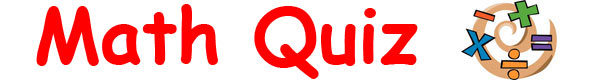Math Quiz for KidsPut your math knowledge to the test with this fun math quiz for kids. Find a wide range of questions & answers related to addition, multiplication, subtraction, division, geometry, numbers and general math knowledge.How many of the twenty questions can you answer correctly? Give it your best shot and find out! When youve finished simply scroll down to the bottom of the page to see how well you did. Sponsored Links

 1. What is the next prime number after 7? 2. The perimeter of a circle is also known as what? 3. 65  43 = ? 4. True or false? A convex shape curves outwards. 5. What does the square root of 144 equal? 6. True or false? Pi can be correctly written as a fraction. 7. What comes after a million, billion and trillion? 8. 52 divided by 4 equals what? 9. What is the bigger number, a googol or a billion? 10. True or false? Opposite angles of a parallelogram are equal. 11. 87 + 56 = ? 12. How many sides does a nonagon have? 13. True or false? -2 is an integer. 14. What is the next number in the Fibonacci sequence: 0, 1, 1, 2, 3, 5, 8, 13, 21, 34, ? 15. 7 x 9 = ? 16. True or false? In an isosceles triangle all sides are unequal. 17. In statistics, the middle value of an ordered set of values is called what? 18. What does 3 squared equal? 19. True or false? -4 is a natural number. 20. 5 to the power of 0 equals what?